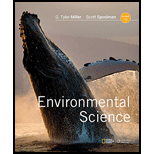Chapter 2, Problem 5CREnvironmental Science (MindTap Cou...

16th Edition
G. Tyler Miller + 1 other
ISBN: 9781337569613

Solutions

Chapter
SectionEnvironmental Science (MindTap Cou...

16th Edition
G. Tyler Miller + 1 other
ISBN: 9781337569613
Textbook Problem

WHAT IS MATTER AND WHAT HAPPENS WHEN IT UNDERGOES CHANGE?What are the two key concepts for this section? What is matter, and what are its three physical states? Distinguish between an element and a compound and give an example of each. What is the periodic table of elements? Define atom and state the atomic theory. Distinguish among protons, neutrons, and electrons. What is the nucleus of an atom? Distinguish between the atomic number and the mass number of an element. What is an isotope? Define molecule and ion and give an example of each. What is acidity? What is pH? Define chemical formula and give two examples.

To determine

The two key concepts of the given section. The meaning of term matter and three physical states of matter. Differences between element and compound with examples. The meaning of periodic table of elements. The meaning of atom and atomic theory. The difference between protons, electron, and neutrons. The meaning of nucleus of an atom. Difference between atomic number and mass number of an element. The meaning of isotope. The meaning of molecule and ion with an example. The meaning of acidity, pH and chemical formula. Two examples of a chemical formula

Explanation

The key concepts of this chapter are as follows:

(i) The matter is made up of the smallest unit called an atom. Combination of atoms produces compounds.

(ii) Atoms are neither created or destroyed whenever matter undergoes a chemical or physical change.

Matter: Anything that has mass and occupies space is called matter. Solid, liquid and gas are three physical states of matter. Matter can exist in these three physical states at a given temperature and pressure.

Difference between element and compound is explained as follows:

 No. Characteristics Element Compound 1. Definition The basic type of matter which has unique properties and cannot be broken down in simple substance by chemical methods is known as an element. A compound consisting of two or more elements which are present in fixed proportions. 2. Example Gold, mercury, silver, copper and many more are examples of elements. For example, carbon dioxide (CO2), water (H2O), sodium chloride (NaCl) and many more

The periodic table of elements: The arrangement of elements based upon their recurring chemical properties in a chart is called the periodic table of elements.

Atom: Atom is the smallest unit of matter which cannot be broken down into smaller units. It is the building block of matter.

Atomic theory: Atomic theory constitutes the idea that all elements are formed from an atom.

Difference between proton, neutron, and electron is explained as follows:

 No. Characteristics Proton Neuron Electron 1. Definition Proton is the subatomic particle which has a positive charge (+) Neutron is the subatomic particle with no change. Neutron is a neutral particle. An electron is a subatomic particle with a negative charge (-) 2. Mass Mass of a proton is 1.67×10-27 kilograms Mass of neutron is also 1.67×10-27 kilograms Mass of electron is 9

Still sussing out bartleby?

Check out a sample textbook solution.

See a sample solution

The Solution to Your Study Problems

Bartleby provides explanations to thousands of textbook problems written by our experts, many with advanced degrees!

Get Started

What is genomics?

Human Heredity: Principles and Issues (MindTap Course List)

1-24 Solve: (9.52104)(2.77105)(1.39107)(5.83102)

Introduction to General, Organic and Biochemistry

Why do distant stars look redder than their spectral types suggest?

Horizons: Exploring the Universe (MindTap Course List)